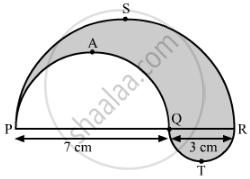# In Fig.5, PSR, RTQ and PAQ are three semicircles of diameters 10 cm, 3 cm and 7 cm respectively. Find the perimeter of the shaded region. - Mathematics

Sum

In PSR, RTQ and PAQ are three semicircles of diameters 10 cm, 3 cm and 7 cm respectively. Find the perimeter of the shaded region. [Use π=3.14]#### Solution

Radius of semicircle PSR = 1/2×10 cm= 5 cm
Radius of semicircle RTQ = 1/2×3 cm = 1.5 cm
Radius of semicircle PAQ = 1/2×7 cm = 3.5 cm

Perimeter of the shaded region = Circumference of semicircle PSR + Circumference of semicircle RTQ + Circumference of semicircle PAQ

= [1/2xx 2π(5) + 1/2xx 2π(1.5) + 1/2xx 2π(3.5)] cm

= π (5 + 1.5 + 3.5) cm

= 3.14 xx 10 cm

= 31.4 cm

Concept: Circumference of a Circle
Is there an error in this question or solution?